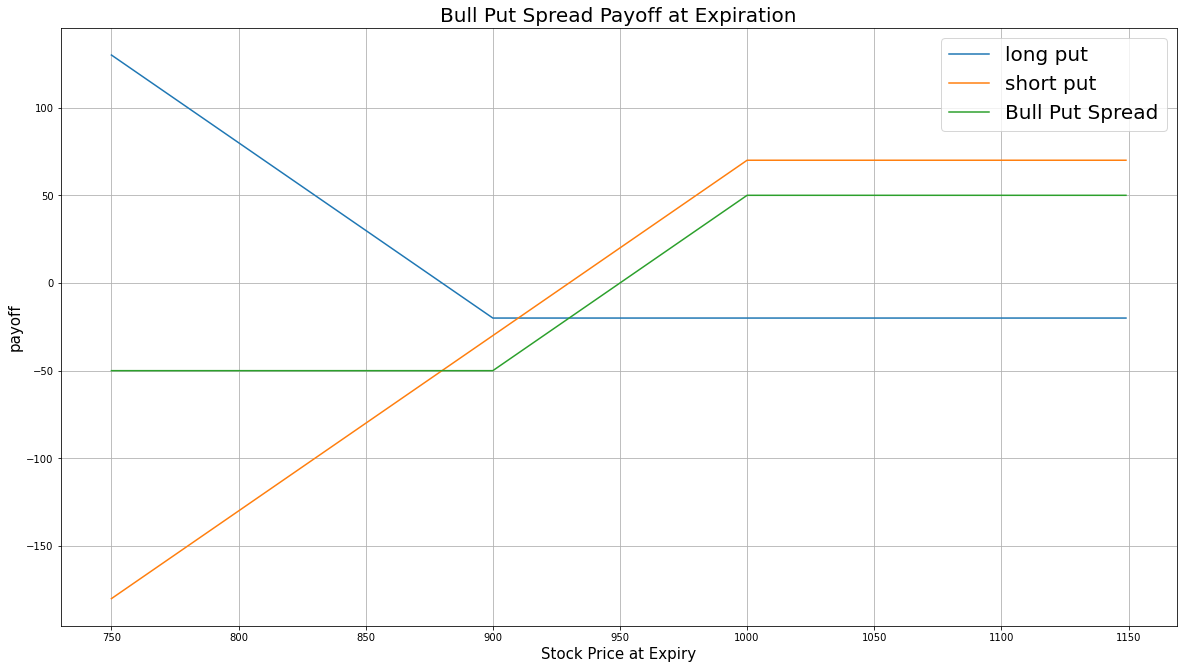# Option Strategies

### Introduction

Bull put spread, also known as long put spread, consists of buying an OTM put and selling an ITM put. Both puts have the same underlying Equity and the same expiration date. The OTM put serves as a hedge for the ITM put. The bull put spread profits from a rise in underlying asset price.

### Implementation

1. In the Initialize method, set the start date, end date, cash, and Option universe.
2. private Symbol _symbol;

public override void Initialize()
{
SetStartDate(2017, 2, 1);
SetEndDate(2017, 3, 5);
SetCash(500000);

_symbol = option.Symbol;
option.SetFilter(universe => universe.IncludeWeeklys()
.Strikes(-15, 15)
.Expiration(TimeSpan.FromDays(0), TimeSpan.FromDays(31)));
}
def Initialize(self) -> None:
self.SetStartDate(2017, 2, 1)
self.SetEndDate(2017, 3, 5)
self.SetCash(500000)

self.symbol = option.Symbol
option.SetFilter(self.UniverseFunc)

def UniverseFunc(self, universe: OptionFilterUniverse) -> OptionFilterUniverse:
return universe.IncludeWeeklys().Strikes(-15, 15).Expiration(timedelta(0), timedelta(31))
3. In the OnData method, select the expiration and strikes of the contracts in the strategy legs.
4. public override void OnData(Slice slice)
{
if (Portfolio.Invested) return;

// Get the OptionChain
var chain = slice.OptionChains.get(_symbol, null);
if (chain.Count() == 0) return;

// Get the furthest expiration date of the contracts
var expiry = chain.OrderByDescending(x => x.Expiry).First().Expiry;

// Select the put Option contracts with the furthest expiry
var puts = chain.Where(x => x.Expiry == expiry && x.Right == OptionRight.Put);
if (puts.Count() == 0) return;

// Select the ITM and OTM contract strikes from the remaining contracts
var putStrikes = puts.Select(x => x.Strike).OrderBy(x => x);
var itmStrike = putStrikes.Last();
var otmStrike = putStrikes.First();
def OnData(self, slice: Slice) -> None:
if self.Portfolio.Invested: return

# Get the OptionChain
chain = slice.OptionChains.get(self.symbol, None)
if not chain: return

# Get the furthest expiration date of the contracts
expiry = sorted(chain, key = lambda x: x.Expiry, reverse=True).Expiry

# Select the put Option contracts with the furthest expiry
puts = [i for i in chain if i.Expiry == expiry and i.Right == OptionRight.Put]
if len(puts) == 0: return

# Select the ITM and OTM contract strikes from the remaining contracts
put_strikes = sorted([x.Strike for x in puts])
otm_strike = put_strikes
itm_strike = put_strikes[-1]
5. In the OnData method, call the OptionStrategies.BullPutSpread method and then submit the order.
6. var optionStrategy = OptionStrategies.BullPutSpread(_symbol, itmStrike, otmStrike, expiry);
Buy(optionStrategy, 1);
option_strategy = OptionStrategies.BullPutSpread(self.symbol, itm_strike, otm_strike, expiry)
self.Buy(option_strategy, 1)

Option strategies synchronously execute by default. To asynchronously execute Option strategies, set the asynchronous argument to Falsefalse. You can also provide a tag and order properties to the Buy method.

Buy(optionStrategy, quantity, asynchronous, tag, orderProperties);

self.Buy(option_strategy, quantity, asynchronous, tag, order_properties)


### Strategy Payoff

This is a limited-reward-limited-risk strategy. The payoff is

$$\begin{array}{rcll} P^{OTM}_T & = & (K^{OTM} - S_T)^{+}\\ P^{ITM}_T & = & (K^{ITM} - S_T)^{+}\\ P_T & = & (P^{OTM}_T - P^{ITM}_T + P^{ITM}_0 - P^{OTM}_0)\times m - fee\\ \end{array}$$ $$\begin{array}{rcll} \textrm{where} & P^{OTM}_T & = & \textrm{OTM put value at time T}\\ & P^{ITM}_T & = & \textrm{ITM put value at time T}\\ & S_T & = & \textrm{Underlying asset price at time T}\\ & K^{OTM} & = & \textrm{OTM put strike price}\\ & K^{ITM} & = & \textrm{ITM put strike price}\\ & P_T & = & \textrm{Payout total at time T}\\ & P^{ITM}_0 & = & \textrm{ITM put value at position opening (credit received)}\\ & P^{OTM}_0 & = & \textrm{OTM put value at position opening (debit paid)}\\ & m & = & \textrm{Contract multiplier}\\ & T & = & \textrm{Time of expiration} \end{array}$$

The following chart shows the payoff at expiration:The maximum profit is the net credit you received when opening the position, $P^{ITM}_0 - P^{OTM}_0$. If the underlying price is higher than the strike prices of both put contracts at expiration, both puts expire worthless.

The maximum loss is $K^{ITM} - K^{OTM} + P^{ITM}_0 - P^{OTM}_0$.

If the Option is American Option, there is a risk of early assignment on the sold contract.

### Example

The following table shows the price details of the assets in the algorithm:

AssetPrice ($)Strike ($)
OTM put5.70767.50
ITM put35.50835.00
Underlying Equity at expiration829.08-

Therefore, the payoff is

$$\begin{array}{rcll} P^{OTM}_T & = & (K^{OTM} - S_T)^{+}\\ & = & (767.50-829.08)^{+}\\ & = & 0\\ P^{ITM}_T & = & (K^{ITM} - S_T)^{+}\\ & = & (835.00-829.08)^{+}\\ & = & 5.92\\ P_T & = & (P^{OTM}_T - P^{ITM}_T + P^{ITM}_0 - P^{OTM}_0)\times m - fee\\ & = & (0-5.92+35.50-5.70)\times100-1.00\times2\\ & = & 2386\\ \end{array}$$

So, the strategy profits \$2,386.

The following algorithm implements a bull put spread strategy:

You can also see our Videos. You can also get in touch with us via Discord.